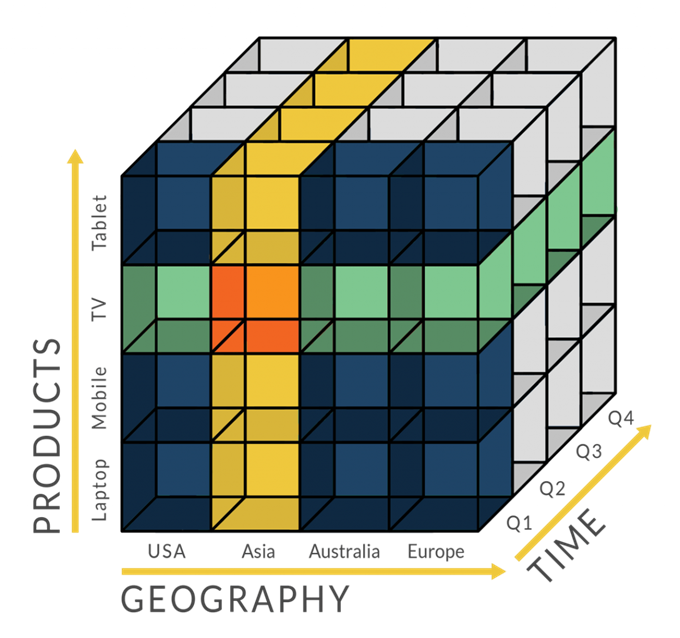# Pandas数据处理简明教程

## Pandas简介

Pandas是python的一个数据分析包，最初由AQR Capital Management于2008年4月开发，并于2009年底开源出来，目前由专注于Python数据包开发的PyData开发team继续开发和维护，属于PyData项目的一部分。Pandas最初被作为金融数据分析工具而开发出来，因此，pandas为时间序列分析提供了很好的支持。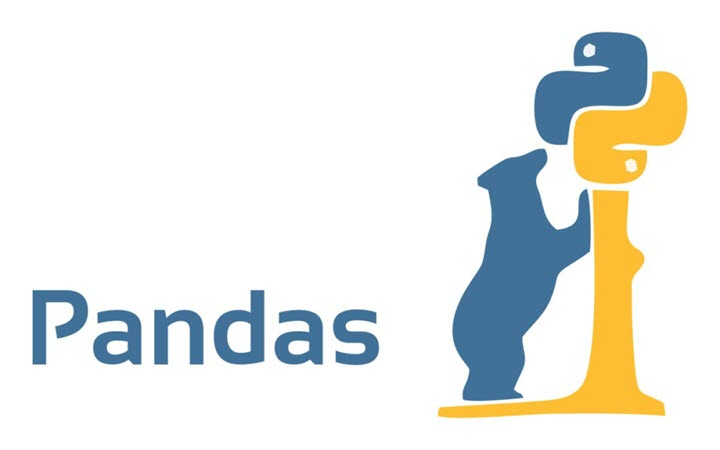Pandas 适用于处理以下类型的数据：

• 与 SQL 或 Excel 表类似的，含异构列的表格数据
• 有序和无序（非固定频率）的时间序列数据
• 带行列标签的矩阵数据，包括同构或异构型数据
• 任意其它形式的观测、统计数据集, 数据转入 Pandas 数据结构时不必事先标记。

Pandas的优势：

• 处理浮点与非浮点数据里的缺失数据，表示为NaN
• 大小可变：插入或删除DataFrame 等多维对象的列
• 自动、显式数据对齐：显式地将对象与一组标签对齐，也可以忽略标签，在 Series、DataFrame 计算时自动与数据对齐
• 强大、灵活的分组（group by）功能：拆分-应用-组合数据集，聚合、转换数据
• 把 Python 和 NumPy 数据结构里不规则、不同索引的数据轻松地转换为 DataFrame 对象
• 基于智能标签，对大型数据集进行切片、花式索引、子集分解等操作
• 直观地合并（merge）、连接（join）数据集
• 灵活地重塑（reshape）、透视（pivot）数据集
• 轴支持结构化标签：一个刻度支持多个标签
• 成熟的 IO 工具：读取文本文件（CSV 等支持分隔符的文件）、Excel 文件、数据库等来源的数据，利用超快的HDF5格式保存/加载数据
• 时间序列：支持日期范围生成、频率转换、移动窗口统计、移动窗口线性回归、日期位移等时间序列功能。

### Series与DataFrame

Pandas 的主要数据结构是Series（一维数据）与DataFrame（二维数据），这两种数据结构足以处理金融、统计、社会科学、工程等领域里的大多数典型用例。Pandas 基于NumPy开发，可以与其它第三方科学计算支持库完美集成。

• Series是一种类似与一维数组的对象，它由一组数据以及一组与之相关的数据便签（即索引）组成，仅由一组数据即可产生最简单的Series。
• DataFrame是一个表格型数据，含有一组有序的列，每一列可以是不同的类型值。DataFrame可以看成是由多个Series组成的字典，它们共用一个索引。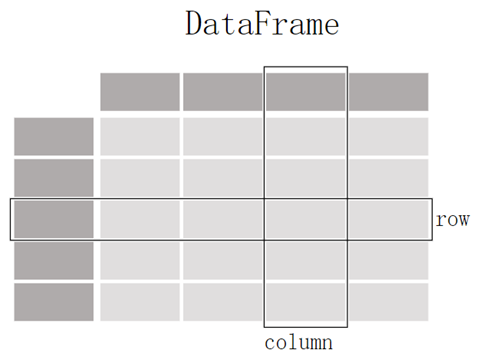• 唯一标识数据，用于定位数据
• 用于数据对齐
• 获取和设置数据集的子集。

>>> df.index
RangeIndex(start=0, stop=3, step=1)

>>>df.columns
Index(['Name', 'Age', 'Sex'], dtype='object')


Pandas库axis=0，axis= 1轴的用法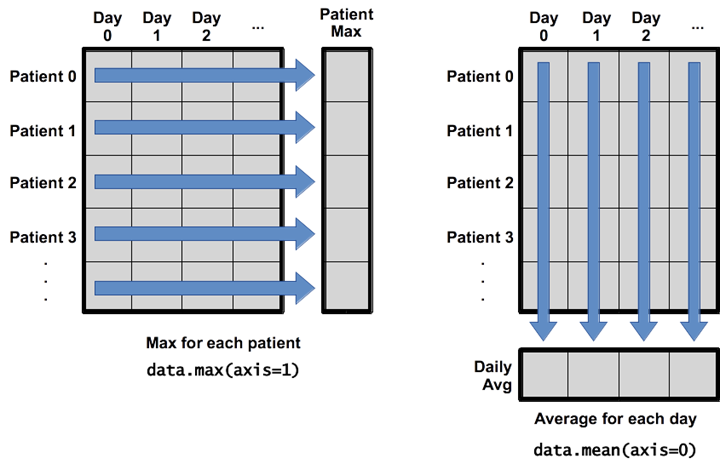• axis = 1：指的是沿着行求所有列的最大值，代表了横轴。
• axis = 0：就是沿着列求所有行的平均值，代表了纵轴。

## Pandas的行、列、索引操作

### Pandas行、列、索引常规操作

print(df.columns)  # 输出列名
df.columns = ['a', 'b', 'c']  # 重命名列名
df = df[['a', 'b', 'c']]  # 只选取想要的列

df = df.transpose()  # 行列转换
df = df.T  # 行列转换的简写方式

df = df.set_index('c')  # 以c列作为索引
df.reset_index() # 重新变为默认的数值索引


### Pandas中的loc和iloc

loc与iloc的区别：

• .loc主要是基于标签(label)的，包括行标签(index)和列标签(columns)，即行名称和列名称，可以使用loc[index_name,col_name]
• .iloc是基于位置的索引，利用元素在各个轴上的索引序号进行选择

# .loc的用法
df.loc  # 选择index为3的一行，这里的’3’是index的名称，而不是序号
df.loc['a']  # 获取index是a的某一行
df.loc[['a', 'b', 'c']]  # 获取index为a,b,c的行
df.loc['c':'h']  # 获取c到h行，包含c和h（左闭右闭）
df.loc[df['A'] > 5]  # 筛选出所有A列>5的行
df.loc[df['A'] > 5, ['C', 'D']]  # 筛选出所有A列>5的行的C和D列

# .iloc的用法
df.iloc # 选择第四行，下标从0开始
df.iloc[[1,3,5]] # 选择第2、4、6行
df.iloc[0:3] # 选择1~3行，和loc不同的是这里是左闭右开
df.iloc[0:3,1:3] # 选择1~3行,2~3列
df.iloc[df['A'] > 5]  # 同上


X = df.iloc[:, :-1]
y = df.iloc[:, -1]


### Pandas与时间序列

# 解析时间格式字符串、np.datetime64、datetime.datetime 等多种时间序列数据。
dti = pd.to_datetime(['1/1/2018', np.datetime64('2018-01-01'), datetime.datetime(2018, 1, 1)])

# 生成 DatetimeIndex、TimedeltaIndex、PeriodIndex 等定频日期与时间段序列。
dti = pd.date_range('2018-01-01', periods=3, freq='H')

# 处理、转换带时区的日期时间数据。
dti = dti.tz_localize('UTC')
dti.tz_convert('US/Pacific')

# 按指定频率重采样，并转换为时间序列。
idx = pd.date_range('2018-01-01', periods=5, freq='H')
ts = pd.Series(range(len(idx)), index=idx)
ts.resample('2H').mean()

# 用绝对或相对时间差计算日期与时间。
friday = pd.Timestamp('2018-01-05')
print(friday.day_name())
saturday = friday + pd.Timedelta('1 day')
print(saturday.day_name())
monday = friday + pd.offsets.BDay() # 添加 1 个工作日，从星期五跳到星期一
print(monday.day_name())


ts['2021'] # 查询整个2021年的
ts['2021-6'] # 查询 2021年6月的
ts['2021-6':'2021-10'] # 6月到10月的
dft['2013-1':'2013-2-28 00:00:00'] # 精确时间
dft['2013-1-15':'2013-1-15 12:30:00']
dft.loc['2013-01-05']


## Pandas的数据类型

Pandas中主要的数据类型有：

• float
• int
• bool
• datetime64[ns]
• datetime64[ns, tz]
• timedelta64[ns]
• timedelta[ns]
• category
• object

 Pandas 类型 Python 类型 NumPy类型 使用场景 object str or mixed string_, unicode_, mixed types 文本或者混合数字 int64 int int_, int8, int16, int32, int64, uint8, uint16, uint32, uint64 整型数字 float64 float float_, float16, float32, float64 浮点数字 bool bool bool_ True/False 布尔型 datetime64[ns] nan datetime64[ns] 日期时间 timedelta[ns] nan nan 两个时间之间的距离，时间差 category nan nan 有限文本值，枚举

df.dtypes  # 各字段的数据类型
df['a'].dtype  # 某个字段的类型
df['a'].astype(float)  # 转换类型


## Pandas数据的获取与处理

import pandas as pd

d = [{'points': 50, 'time': '5:00', 'year': 2010},
{'points': 25, 'time': '6:00', 'month': "february"},
{'points': 90, 'time': '9:00', 'month': 'january'},
{'points_h1': 20, 'month': 'june'}]

df = pd.DataFrame(d)


## Pandas用于探索数据

### 查看数据基本情况

• tail(n)：查看DataFrame对象的最后n行
• shape()：查看行数和列数
• info()：查看索引、数据类型和内存信息
• describe()：查看数值型列的汇总统计
• describe(include=[np.number]) #指定数字类型
• describe(include=[np.object]) #指定object类型
• describe(include=[‘category’]) #指定列名
• value_counts(dropna=False)：查看Series对象的唯一值和计数
• apply(pd.Series.value_counts)：查看DataFrame对象中每一列的唯一值和计数
• unique()：返回唯一值
• corr()：返回列与列之间的相关性
DataFrame.corr(method='pearson', min_periods=1)

• method：可选值为{‘pearson’, ‘kendall’, ‘spearman’}
• min_periods：样本最少的数据量

### 异常数据的处理

Pandas中存在两个检查空值的方法，pandas.DataFrame.isna() 和 pandas.DataFrame.isnull()，两个方法使用起来我完全一致。

• isna().sum()

• replace(‘-‘,’np.nan’)：用Null值替换’-‘
• replace(1,’one’)：用‘one’代替所有等于1的值
• replace([1,3],[‘one’,’three’])：用’one’代替1，用’three’代替3

DataFrame.dropna(axis=0, how='any', thresh=None, subset=None, inplace=False)

• axis：它决定轴是行还是列。
• 如果它是 0 或’index’，那么它将删除包含缺失值的行。
• 如果它是 1 或’column’，那么它将删除包含缺失值的列。默认情况下，它的值是 0
• how：这个参数决定函数如何删除行或列。它只接受两个字符串，可以是 all 或 all。默认情况下，它被设置为 any。
• any – 如果行或列中有任何空值，就会删除它。
• all – 如果行或列中缺少所有值，则放弃该行或列
• thresh：它是一个整数，指定了防止行或列丢失的非缺失值的最少数量
• subset：它是一个数组，其中有行或列的名称，用于指定删除程序
• inplace：它是一个布尔值，如果设置为 True，将就地改变调用者 DataFrame。默认情况下，它的值是 False

• dropna()：删除所有包含空值的行
• dropna(axis=1)：删除所有包含空值的列
• dropna(axis=1,thresh=n)：删除所有小于n个非空值的行

DataFrame.fillna(value=None, method=None, axis=None, inplace=False, limit=None, downcast=None)

• value: 用于填充空值的值
• axis: 填充缺失值所沿的轴
• inplace: boolean, 默认为False。若为True， 在原地填满
• limit: int, 默认为None， 如果指定了方法， 则这是连续的NaN值的前向/后向填充的最大数量
• downcast: dict, 默认None， 字典中的项为类型向下转换规则。

• fillna(x)：用x替换DataFrame对象中所有的空值
• fillna(s.mean())：用均值填充
• fillna(s.median())：用中位数填充

DataFrame.drop_duplicates(subset=None, keep='first', inplace=False, ignore_index=False)

• subset： 输入要进行去重的列名，默认为None
• keep： 可选参数有三个：‘first’、 ‘last’、 False， 默认值 ‘first’。其中，
• first表示： 保留第一次出现的重复行，删除后面的重复行。
• last表示： 删除重复项，保留最后一次出现。
• False表示： 删除所有重复项。
• inplace：布尔值，默认为False，是否直接在原数据上删除重复项或删除重复项后返回副本。

df.drop_duplicates(subset=['A','B'],keep='first',inplace=True)

### 数据的汇总统计

Pandas中常用的统计函数：

• .count() #非空元素计算
• .size() #包含NaN的计数
• .min() #最小值
• .max() #最大值
• .idxmin() #最小值的位置，类似于R中的min函数
• .idxmax() #最大值的位置，类似于R中的max函数
• .quantile(0.1) #10%分位数
• .sum() #求和
• .mean() #均值
• .median() #中位数
• .mode() #众数
• .var() #方差
• .std() #标准差
• .skew() #偏度
• .kurt() #峰度

import pandas as pd
import numpy as np

def status(x):
return pd.Series(
[x.count(), x.min(), x.idxmin(), x.quantile(.25), x.median(), x.quantile(.75), x.mean(), x.max(), x.idxmax(),
index=['总数', '最小值', '最小值位置', '25%分位数', '中位数', '75%分位数', '均值', '最大值', '最大值位数', '平均绝对偏差', '方差', '标准差', '偏度',
'峰度'])

if __name__ == "__main__":
# df = pd.DataFrame(status(d1))
df = pd.DataFrame(np.array([d1, d2, d3]).T, columns=['x1', 'x2', 'x3'])
df.apply(status)


Pandas中的分组groupby()

df.groupby(by=['col1','col2']).size()

df.groupby(by=['col1', 'col2']).size().reset_index(name='counts')

groupby结合agg进行聚合：

df[['col1', 'col2', 'col3', 'col4']].groupby(['col1', 'col2']).agg(['mean', 'count'])

SELECT col1, col2, avg(col1), count(col2) FROM df GROUP BY col1, col2


key1 = df.groupby(["key1"], as_index=False)["data1"].agg({"col_name": "count"})

Pandas中的排序sort_values()

• sort_values(col1)：按照列col1排序数据，默认升序排列
• sort_values(col2, ascending=False)：按照列col1降序排列数据
• sort_values([col1,col2], ascending=[True,False])：先按列col1升序排列，后按col2降序排列数据

Pandas Dataframe的遍历

for index, row in df.iterrows():
print(row['c1'], row['c2'])

## Pandas的apply函数

Pandas 的 apply() 方法是用来调用一个函数（Python method），让此函数对数据对象进行批量处理。

DataFrame.apply(func, axis=0, raw=False, result_type=None, args=(), **kwargs)

• func：function 应用于每个列或行的函数。
• axis：{0 或 ‘index’, 1 或 ‘columns’}, 默认为 0
• 0或‘index’：将功能应用于每列。
• 1或‘columns’：将功能应用于每一行。
• raw：确定是否将行或列作为Series或ndarray对象传递：
• False：将每个行或列作为 Series 传递给该函数。
• True：传递的函数将改为接收ndarray对象。如果您仅应用NumPy缩减功能，则将获得更好的性能。
• ‘expand’：list-like结果将变成列。
• ‘reduce’：如果可能，则返回一个序列，而不是扩展list-like的结果。这与‘expand’相反。

ser.apply(fun) # 自定义
ser.apply(max) # python 内置函数
ser.apply(lambda x: x*2) # lambda
ser.apply(np.mean) # numpy 等其他库的函数 ufunc
ser.apply(pd.Series.first_valid_index) # Pandas 自己的函数
ser.apply('count') # Pandas 自己的函数
ser.apply('shape') # Pandas 自己的属性
ser.apply('tan') # numpy 的 ufunc 名
# 多个函数
ser.apply([sum, 'count']) # 相当于 .aggregate, 即.agg
ser.apply(np.array(["sum", "mean"]))
ser.apply({'Q1':sum, 'Q2':'count'}) # 同上
#SQL中row_number()的实现
df['Rank'] = df.groupby(by=['交易日期'])['交易时间'].apply(lambda x: x.rank(ascending=False))


## Pandas平移函数shift()与diff()

### shift()函数

shift()函数主要的功能就是使数据框中的数据移动，若freq=None时，根据axis的设置，行索引数据保持不变，列索引数据可以在行上上下移动或在列上左右移动；若行索引为时间序列，则可以设置freq参数，根据periods和freq参数值组合，使行索引每次发生periods*freq偏移量滚动，列索引数据不会移动。

DataFrame.shift(periods=1, freq=None, axis=0, fill_value=<no_default>)

• period：表示移动的幅度，可以是正数，也可以是负数，默认值是1,1就表示移动一次，注意这里移动的都是数据，而索引是不移动的，移动之后没有对应值的，就赋值为NaN。
• freq： DateOffset, timedelta, or time rule string，可选参数，默认值为None，只适用于时间序列，如果这个参数存在，那么会按照参数值移动时间索引，而数据值没有发生变化。
• axis： {0, 1, ‘index’, ‘columns’}，表示移动的方向，如果是0或者’index’表示上下移动，如果是1或者’columns’，则会左右移动。
• fill_value：空行需要填充的数值

### diff()函数

DataFrame.diff(periods=1, axis=0)

• periods：移动的幅度，int类型，默认值为1。
• axis：移动的方向，{0 or ‘index’, 1 or ‘columns’}，如果为0或者’index’，则上下移动，如果为1或者’columns’，则左右移动。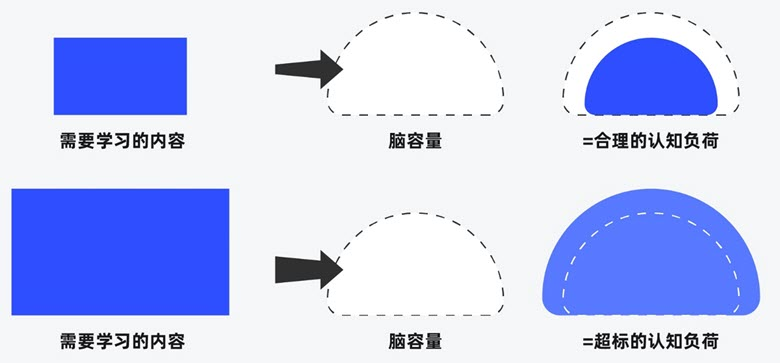##### 界面设计和游戏设计中的认知负荷##### 理解认知偏差